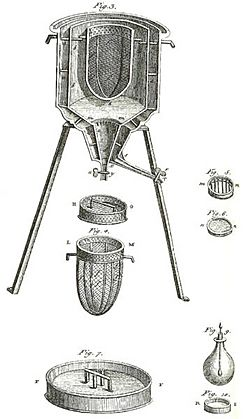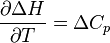# Thermochemistry facts for kids

Kids Encyclopedia Facts

Thermochemistry is the study of the energy and heat to do with chemical reactions and physical transformations (physical changes). Physical transformations are when a state of matter (a solid or liquid, for example) changes to another state. Examples of transformations include melting (when a solid becomes a liquid) and boiling (when a liquid becomes a gas).

A reaction gives out or takes in energy. A physical transformation also gives out or takes in energy. Thermochemistry looks at these energy changes, particularly on a system's energy exchange with its surroundings. Thermochemistry is useful in predicting reactant and product quantities at all times during a given reaction. Thermochemists do this by using data, including entropy determinations. Thermochemists will tell if a reaction is spontaneous or non-spontaneous, favorable or unfavorable.

Endothermic reactions take in heat. Exothermic reactions give out heat. Thermochemistry combines the concepts of thermodynamics with the idea of energy in the form of chemical bonds. It includes calculations of such quantities as heat capacity, heat of combustion, heat of formation, enthalpy, entropy, free energy, and calories.The world’s first ice-calorimeter, used in the winter of 1782-83, by Antoine Lavoisier and Pierre-Simon Laplace. It was used to find the heat evolved in various chemical changes. These calculations were based on Joseph Black’s earlier discovery of latent heat. These experiments started thermochemistry.

## History

Thermochemistry started with two ideas:

1. Lavoisier and Laplace’s law (1780): The energy change for any transformation is equal and opposite to energy change for the reverse process.
2. Hess' law (1840): The energy change for any transformation is the same whether it goes in one step or many.

These discoveries came before the first law of thermodynamics (1845). They helped scientists understand this law.

Edward Diaz and Hess investigated specific heat and latent heat. Joseph Black developed the concept of latent energy changes.

Gustav Kirchhoff showed in 1858 that change in the heat of reaction is given by the difference in heat capacity between products and reactants:${{\partial \Delta H}\over\partial T} = \Delta C_p$. Integrating this equation allows the evaluation of the heat of reaction at one temperature from measurements at another temperature.

## Calorimetry

The measurement of heat changes is called calorimetry. It measures the heat of chemical reactions or physical changes. A calorimeter, a device for calorimetry, is usually a closed in chamber.

Calorimetry has these steps: Chemists make the change happen inside the chamber. The temperature of the chamber is measured either using a thermometer or thermocouple. The temperature is plotted against time to give a graph. Chemists use the graph to calculate fundamental quantities.

Modern calorimeters have small computers that measure the temperature and give the calculated data quickly. One example is a differential scanning calorimeter (DSC).

## Systems

Several thermodynamic definitions are very useful in thermochemistry. A "system" is the specific section of the universe that is being studied. Everything outside the system is considered the surrounding or environment. A system may be:

• an isolated system — when it cannot exchange energy or matter with the surroundings, as with an insulated bomb calorimeter;
• a closed system — when it can exchange energy but not matter with the surroundings, as with a steam radiator;
• an open system — when it can exchange both matter and energy with the surroundings, a pot of boiling water, for example.

## Processes

A system undergoes a "process" when one or more of its properties (characteristics) changes. A process relates (connects) to the change of state. An isothermal (same temperature) process happens when the temperature of the system stays the same. An isobaric (same pressure) process happens when the pressure of the system stays the same. An adiabatic (no heat exchange) process happens when no heat moves around.

## Related pages

• Important publications in thermochemistry
• Isodesmic reaction
• Principle of maximum work
• Reaction Calorimeter
• Thomsen-Berthelot principle
• Julius Thomsen
• Thermodynamic databases for pure substances
• Calorimetry
• Thermal physicsThermochemistry Facts for Kids. Kiddle Encyclopedia.